#### You may also like### I'm Eight

Find a great variety of ways of asking questions which make 8.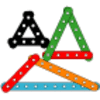### Let's Investigate Triangles

Vincent and Tara are making triangles with the class construction set. They have a pile of strips of different lengths. How many different triangles can they make?### Noah

Noah saw 12 legs walk by into the Ark. How many creatures did he see?

# Number Round Up

##### Age 5 to 7 Challenge Level:

If you are a teacher, click here for a version of the problem suitable for classroom use, together with supporting materials. Otherwise, read on ...

Arrange the numbers $1$ to $6$ in each set of circles below.

The sum of each side of the triangle should equal the number in the centre of the triangular shape.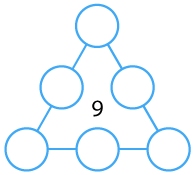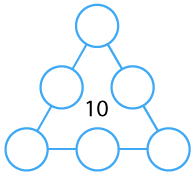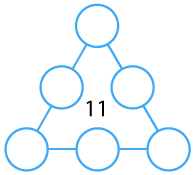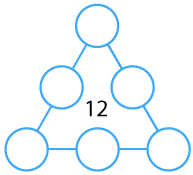Once you've had a chance to think about it, click below to see how three different pupils began working on the task.

Dan said:

"I used counters which had $1$ to $6$ on them.

I put the counters in a triangle in any old way, then I added up the sides.

Then I moved the counters around to try and get the right total on each side."

Emma said:

"I noticed that three of the numbers are odd ($1, 3$ and $5$) and three of the numbers are even ($2, 4$ and $6$).  I thought this might help.

I know that $9$ is an odd number so it can be made using odd + odd + odd or using even + even + odd."

Farah said:

"If I want a small total on each side, I'll need small numbers in the corners of the triangle."

Can you take each of these starting ideas and develop it into a solution?

This activity originally featured in the hands-on Brain Buster Maths Boxes, developed by members of the NRICH Team and produced by BEAM. These resources are out of print but can still be found on Amazon.